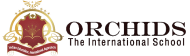Enquire Now

# Percentage Calculator

What is
% of

is what % of

is
% of what?
What Is a Percentage Calculator?
A percentage calculator is a tool to calculate the percentage of a value. This tool makes it easy to calculate the percentage with only two values. The two values are the total number and the number of out of the total.
How to Find Percentage on Calculator?
The online percentage calculator gives you ready access to a calculator to find the percentage of a value or number. The following steps could be followed to calculate a percentage on the percentage calculator tool.
Step 1: Type the number for each input field.
Step 2: Click on the enter button to get the exact percentage.
Percentage Calculation Formula
The percentage formula is calculated when the value is divided by the total which is multiplied by a hundred. In order to manually calculate the percentage, input your values in the formula given below. Percentage Formula: Value * 100 / Total Value
How to Calculate the Percentage of a Number?
The percentage can be calculated when you have two numbers that you need to calculate.
For example: Let’s say you want to calculate the percentage of the value 5 out of the total value 10.
Percentage Formula = 5/10 * 100= 50%
Hence the value 5 is 50 percent of the total number ten.
How to Calculate Profit Percentage?
The profit percentage is calculated to learn about the profit made after a product is sold. For instance, the shopkeeper bought a table for Rs 1200 and later sold it to a customer for Rs. 2600.
Example:
Selling price= Rs. 1200; Cost price= Rs. 2600.
Since the selling price is less than the cost price.
Profit=1200 - 1600= Rs. 400
Using percentage formula = (Profit / Cost price) * 100
=400/1200 * 100
=33.33%
The profit made by the shopkeeper was 33.33 percent more than the selling price.
How to Calculate Average Percentage?
In order to calculate the percentage, you need at least two values. If you calculate the average percentage of a number, then you need to use two or more values to fit in the formula below.
Example:Let’s say you want to calculate the percentage of the marks Rajesh scored in three subjects on the term exam. He scored 55 percent in Maths, 60 percent in Science and 80 percent in English. How would you calculate the average percentage of the marks Rajesh scored?
( Note: The percentage indicated for each subject is written in the percentage of the marks scored and not the marks.)
Solution:
• Maths= 55 %
• Science= 60%
• English=80%
Sum all the percentages that you need to calculate the average percentage for and divide it by the total number of subjects.
• Calculate Average Percentage=(55+60+80)/3
• =195/3
• =65%
Hence, the average score Rajesh scored in the three subjects is 65%.
You can use the percentage calculator to check if you have calculated the correct percentage.

| K12 Techno Services ®

ORCHIDS - The International School | Terms | Privacy Policy | Cancellation